Function Repository Resource:

# StauduharGaloisGroup

Use Stauduhar's method for Galois groups of polynomials

Contributed by: Tessa Wildsmith (additional contributions by Wolfram Staff)
 ResourceFunction["StauduharGaloisGroup"][poly,var] uses Stauduhar's method to find the Galois group for a polynomial poly in the variable var. ResourceFunction["StauduharGaloisGroup"][poly,var,σ,tol] find the Galois group with ordering of the roots σ with precision tol.

## Details

This is restricted to polynomials with integer coefficients and of degree no larger than 10.
Stauduhar's method is an algorithm to find the Galois group of any monic irreducible integer polynomial.
For third-degree polynomial, the result includes:
 "Sigma" the ordering of the roots "Tolerance" high precision approximation "Roots" the roots of the polynomial "Discriminant" the discriminant "SquareRootDiscriminant" the square root of the discriminant "GaloisGroup" the Galois group
Additional information is provided for higher degrees.
Degree 4:
 "Right cosets of Dh4 in S4" function for "Right cosets of Z4 in Dh4" original function for CyclicGroup "Trimmed function for Z4" trimmed function for CyclicGroup
Degree 5:
 "RightCosetsF" right cosets of F "Original function for F20" original function for permutation group F20 "Trimmed function for F20" trimmed function for permutation group F20 "Original function for Z5" original function for CyclicGroup "Trimmed function for Z5" trimmed function for CyclicGroup
Degree 6:
 "RightCosetsDh" right cosets of "RightCosetsS" right cosets of "RightCosetsZ" right cosets of CyclicGroup "RightCosetsG1" right cosets for permutation group G1 "RightCosetsG2" right cosets for permutation group G2 "RightCosetsG" right cosets for permutation group G
Degree 7:
 "RightCosetsDh" right cosets of "RightCosetsG" right cosets for permutation group G "RightCosetsZ" right cosets of CyclicGroup
Stauduhar's method starts with an initial ordering σ for the roots of a polynomial. The method is reliant upon high-precision approximations of the roots for each given polynomial. The method uses invariant functions; in order to isolate the Galois group, high-precision results are required to correctly identify integer results. If it yields no integer results, then the search is continued by testing conjugate values on a transitive subgroup decision tree. The routines hinged upon defining all of the transitive subgroups of S8, S9 and S10.

## Examples

### Basic Examples (8)

Third-degree polynomial:

 In:=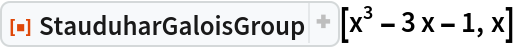Out=Show only the roots:

 In:=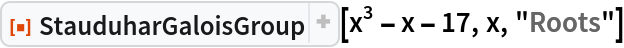Out=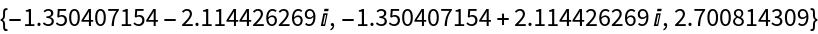Fourth-degree polynomial:

 In:=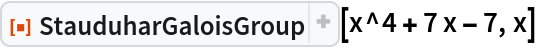Out=Fifth-degree polynomial:

 In:=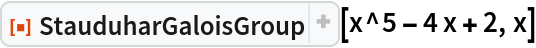Out=Sixth-degree polynomial:

 In:=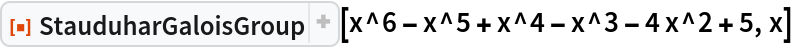Out=Seventh-degree polynomial:

 In:=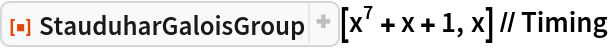Out=Eighth-degree polynomial:

 In:=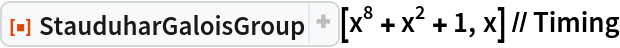Out=Ninth-degree polynomial:

 In:=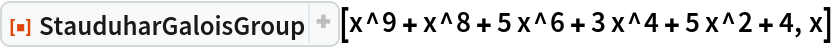Out=Tenth-degree polynomial:

 In:=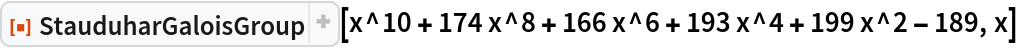Out=### Possible Issues (1)

Stauduhar method works only for polynomials with integer coefficients: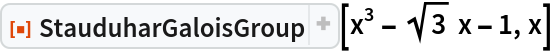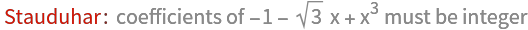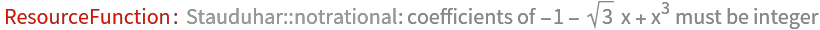Out=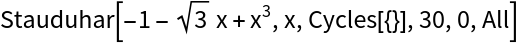Enrique Zeleny

## Version History

• 1.0.0 – 08 November 2021